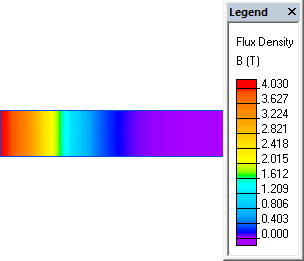QuickField

A new approach to field modelling

Main >> Applications >> Sample problems >> Transient eddy currents in a semi-infinite solid

# Transient eddy currents in a semi-infinite solid

QuickField simulation example

This is an example of the transient eddy currents simulation, performed with QuickField software. The surface of semi-infinite solid plate is suddenly subjected to a constant magnetic potential A0.

Problem Type
Plane-parallel problem of transient magnetics.

Geometry

Given
Magnetic permeability of material μ=1;
Conductivity of material g=2.5 MS/m;

Determine current distribution within the conductor.

Solution
The model length 20 m is arbitrarily selected such that no significant potential change occurs at the end points for the time period of interest. The final time of 0.25 s is sufficient for the theoretical response comparison. A time step of 0.005 s is used.

Results
Flux density distribution in a semi-infinite solid body:Graph of flux density distribution in a semi-infinite solid body:QuickField ANSYS Theory Time t = 0.15 s Coordinate x,m Vector Potential A, Wb/m 0.2517 0.822 0.831 0.831 0.4547 0.280 0.278 0.282 0.6914 0.053 0.044 0.05 Flux Density B, T 0.2517 3.692 3.687 3.707 0.4547 1.716 1.794 1.749 0.6914 0.418 0.454 0.422 Eddy Current Density j, A/mm² 0.2517 -8.06 -7.80 -7.77 0.4547 -6.56 -6.77 -6.63 0.6914 -2.34 -2.45 -2.43